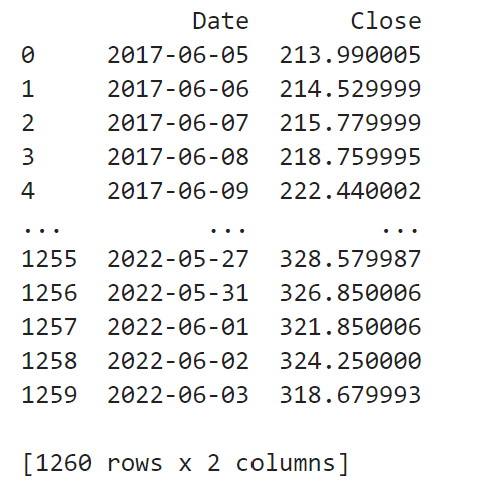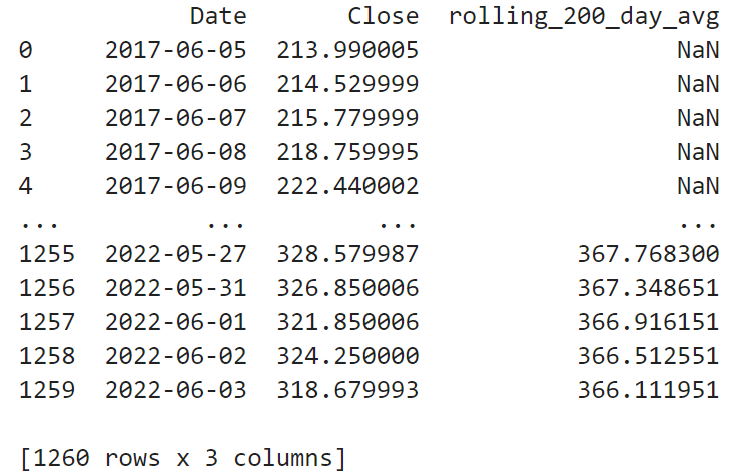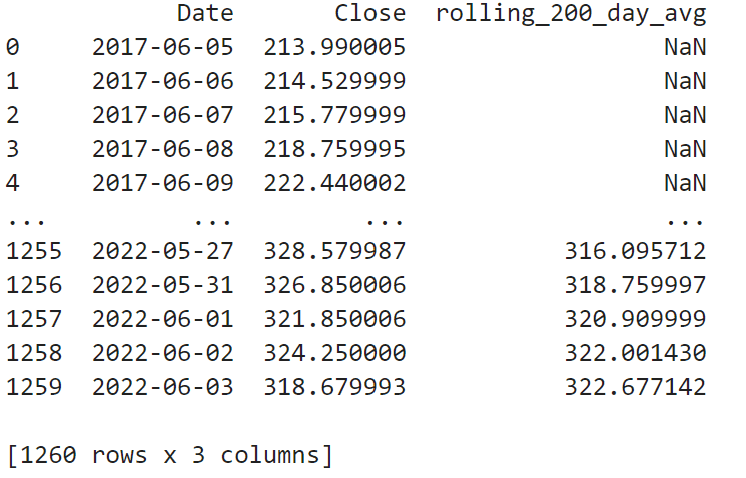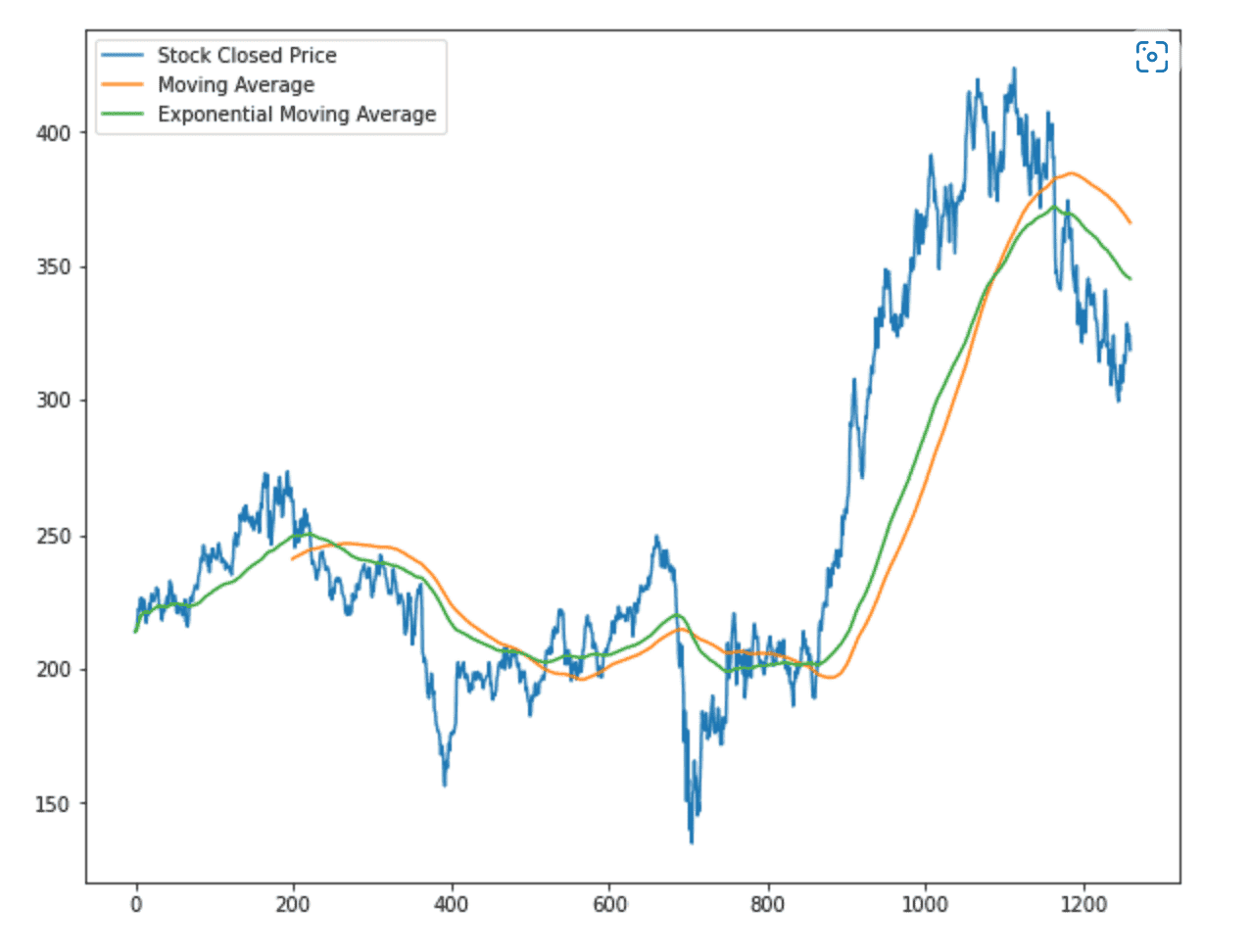# Neural Networks : Which stock has the biggest increase ?

• MHB
• mathmari

#### mathmari

Gold Member
MHB
Hey!On 20.06.2022 we have 1 million dollars for 10 days, so until 30.06.2022, and we want to increase the capital through shares.
(The data of the shares can be found on finance.yahoo.com)

So we want to check which stock has the biggest increase in this period.
So we have to use the final price of each stock and predict what it will do in the said period. So we need to train a neural network with suitable input data.

Do we have to do the following steps ?

1) We are looking for data of the last 5 years on the page.

2) We use moving averages. So to give a value to a day we look a few days before and add all the days and calculate the average. For example, we go 200 days before.
But what kind of moving average do we use? The exponentially smoothed average?

3) We form the input data so that the neural network finds the answer.

4) Of data motives, we look for the 80% for the training and the 20% for the confirmation.

5) So we take all the data of the stocks until 15.06.2022 and train the network. Then we use the weights and start with the forecast for each share from 16.06.2022 to 30.06.2022.

6) We find which stock has the biggest increase.

Is this the right approach?

Could you explain to me in more detail what all this looks like in practice? For example, in step 3, how do we form the input data so that the network can give us an answer? :unsure:

Trying to forecast stock movement is the ultimate problem of financial markets. It isn't possible without some extreme niche scenario and proprietary data (in my opinions and from everyone I know who works in this field). This problem has some great qualities that we want when modeling: a huge amount of historical data to train on, we only need to forecast one day into the future which means we will be able to check immediately how the model is doing, and the financial impact of a successful model would be like printing money. The reason why this is too good to be true is in the day-to-day time span stocks are a random walk. In a longer time horizon (10+ years) a stock will go up if the company is relatively successful, but when you zoom into the daily level you'll see that random noise dominates the day-to-day movement and models will not be accurate over time even when trying to predict up/down from yesterday's price.

All of that said...your approach is good from a predictive modeling perspective.
• 5 years of data is great.
• This will be a time series structure so we definitely want to calculate moving averages, lags, and rolling stats for various time windows. It is common to use a variety of time windows for averages like "past day", "past 7 days", "past 30 days", etc. This helps capture complex trends across different time frames that a single value can't capture.
• The algorithm and model type we use isn't critical. You can use a neural network or you could use a linear model. The best method will depend on the data and scenario. More complex isn't always better.
• To train/validate it's key to split on dates, so you would train on the first 80% of the days available then predict the next 20% of days to evaluate performance. This topic is more nuanced than that in reality, but this is a good start. It's critical to not split the data into a random 80/20 split. It has to be split by date

• 5 years of data is great.

So do we download from the mentioned site the data of the last 5 years for each stock? :unsure:

• This will be a time series structure so we definitely want to calculate moving averages, lags, and rolling stats for various time windows. It is common to use a variety of time windows for averages like "past day", "past 7 days", "past 30 days", etc. This helps capture complex trends across different time frames that a single value can't capture.

Could you explain that point further? So do we calculate various averages? :unsure:

• The algorithm and model type we use isn't critical. You can use a neural network or you could use a linear model. The best method will depend on the data and scenario. More complex isn't always better.

Since the exercise asks for a neural network we should use this one, shouldn't we? :unsure:

• To train/validate it's key to split on dates, so you would train on the first 80% of the days available then predict the next 20% of days to evaluate performance. This topic is more nuanced than that in reality, but this is a good start. It's critical to not split the data into a random 80/20 split. It has to be split by date

So the first 80% of the days are used for the training and the next 20% for the validation, right? How exactly does this work? :unsure:

So do we download from the mentioned site the data of the last 5 years for each stock? :unsure:
5 years isn't necessary but it's a good length. For annual time series models it's good to have at least 2 full years to observe the entire cycle multiple times. If you only have 6 months for example, it's very likely the model will not forecast well for the other 6 months of the year not seen. So if you have 5 years I would recommend using it. If it's less, use as much as possible.

Could you explain that point further? So do we calculate various averages? :unsure:
Sure. So let's say you only use the rolling average of the last 200 days. This feature will not change much when the stock price jumps up/down in a single day. If the average is 100 today and the stock jumps to 130 tomorrow, the 200 day rolling average won't move much and wouldn't capture this important detail. If you only use the last 7 days then the model could miss out of longer trends over 30 days, 90 days, etc. For this reason it's common to use a variety of windows and test which of them show up as important to a model. It's something that requires a bit of guess and check but you can easily make these features in Python.

Since the exercise asks for a neural network we should use this one, shouldn't we? :unsure:
If that's what is asked, then that's the way to go. Neural network can mean a large variety of things though. There are many different network structures and types of data that they work with, so I would look to see if any hints about this are given. If you are using a simple feed-forward network you still need to decide how many layers to use and the dimensions of each layer. This topic can get quite dense and complex, so hopefully you have some direction of how to start from your exercise?

So the first 80% of the days are used for the training and the next 20% for the validation, right? How exactly does this work? :unsure:
If you use Python to load your data, you should have a date column for each row. I would use sklearn's TimeSeriesSplit to make it easy. If you want to try manually then it's not hard to code. Sort your data by date then grab the first 80% of rows for model training and the later 20% of rows for testing. With time series data, the most recent data points are usually the most important. If a model does well for 2018-2021 but is off in 2022, we should focus on 2022 and not the average over all years.

5 years isn't necessary but it's a good length. For annual time series models it's good to have at least 2 full years to observe the entire cycle multiple times. If you only have 6 months for example, it's very likely the model will not forecast well for the other 6 months of the year not seen. So if you have 5 years I would recommend using it. If it's less, use as much as possible.

So we go at the sitye and we search for the stocks that we are interested in, for example Amazon (AMZN) At time period what do we select? The dates 04/06/2017 till 04/06/2022 or do we not choose the date of today? And which frequency do we choose? Daily/Weekly/Monthly? And at Show? Historical Prices or something else? Having chosen all the filters then do we download this file? :unsure:

Sure. So let's say you only use the rolling average of the last 200 days. This feature will not change much when the stock price jumps up/down in a single day. If the average is 100 today and the stock jumps to 130 tomorrow, the 200 day rolling average won't move much and wouldn't capture this important detail. If you only use the last 7 days then the model could miss out of longer trends over 30 days, 90 days, etc. For this reason it's common to use a variety of windows and test which of them show up as important to a model. It's something that requires a bit of guess and check but you can easily make these features in Python.

So we calculate various averages, of the last day, of the last 7 days, of the last 30 days, of the last 200 days, right? And how do we see that we have capture the important details? With a graph? :unsure:

So we go at the sitye and we search for the stocks that we are interested in, for example Amazon (AMZN) At time period what do we select? The dates 04/06/2017 till 04/06/2022 or do we not choose the date of today? And which frequency do we choose? Daily/Weekly/Monthly? And at Show? Historical Prices or something else? Having chosen all the filters then do we download this file? :unsure:
That really all depends on the scenario or the assignment. You could make a model for hour, daily, weekly, or monthly. Usually it would be hourly or daily though in my experience. If you are trying to forecast the stock price for tomorrow then I would try daily first.

So we calculate various averages, of the last day, of the last 7 days, of the last 30 days, of the last 200 days, right? And how do we see that we have capture the important details? With a graph? :unsure:
You will need to make these rolling averages though, which is important. For a rolling 30 day average, on row 31 it will average 1-30. On day 32 it will average 2-31. It always looks back N days from the current date.

For feature importance there are different methods of calculating this, but I like permutation importance.

That really all depends on the scenario or the assignment. You could make a model for hour, daily, weekly, or monthly. Usually it would be hourly or daily though in my experience. If you are trying to forecast the stock price for tomorrow then I would try daily first.

So we download the csv file and then we write the following code to get the columns of Date and Close, since we want only these ones to calculate the moving average, right?

Code:
import pandas as pd

print(df)

Then we get :From these information do we take 5 days and the 6th one as target, then the next 5 days and the next one as a target again and so on? :unsure:

Last edited by a moderator:
Looks good so far. I would use the rolling method from pandas. From your screenshot you can type in:
Code:
df['rolling_7_day_avg'] = df.rolling(7).mean()

Looks good so far. I would use the rolling method from pandas. From your screenshot you can type in:
Code:
df['rolling_7_day_avg'] = df.rolling(7).mean()

I have read that the most popular moving average in finance issues is with 200 days, is that correct? So if we used this moving average we would replace in your command "7" with "200" ?
Or should we use various windows, i.e. 7, 30, 200 and check which is better?

I added your command in my code and I got the below error :

Code:
C:\Users\...\AppData\Local\Temp\ipykernel_608\464235909.py:7: FutureWarning: Dropping of nuisance columns in rolling operations is deprecated; in a future version this will raise TypeError. Select only valid columns before calling the operation. Dropped columns were Index(['Date'], dtype='object')
df['rolling_7_day_avg'] = df.rolling(7).mean()

What does this mean?

:unsure:

Sure, try 200 if that's what you think could be useful. :)

Hmm, maybe try this?
Code:
f['rolling_200_day_avg'] = df.rolling['Close'](200).mean()

Sure, try 200 if that's what you think could be useful. :)

Hmm, maybe try this?
Code:
f['rolling_200_day_avg'] = df.rolling['Close'](200).mean()

I get :So does this mean that at the first 200 lines at the third column we have NaN ? Do we need the moving average to construct the neural network ? What about the values with NaN ? :unsure:

When we use 7 days instead of 200 we get :Are these averages more close to the original values of "Close" ? Does this mean that between these two the option "7 days" is better ? :unsure:

Last edited by a moderator:
If you want to calculate the 200 day average you need 200 datapoints, so the first 199 rows won't have a value. For 7 day average, rows 1-6 won't. To deal with this usually we can start training the model after these values have had time to populate. You could start training your model in January 2018 for example to make it a nice round starting point.

Does it make sense why you see the NaN's? It's a definition of the N_day_average needing N points to be a coherent value. Less than N is not averaging N datapoints.

If you want to calculate the 200 day average you need 200 datapoints, so the first 199 rows won't have a value. For 7 day average, rows 1-6 won't. To deal with this usually we can start training the model after these values have had time to populate. You could start training your model in January 2018 for example to make it a nice round starting point.

Does it make sense why you see the NaN's? It's a definition of the N_day_average needing N points to be a coherent value. Less than N is not averaging N datapoints.

I see! What do you mean by "start training your model in January 2018 for example to make it a nice round starting point" ? How do check which number of day average os the best one? I have read that 200 is the best one, but how do we see that in praxis? :unsure:

Last edited by a moderator:
• To train/validate it's key to split on dates, so you would train on the first 80% of the days available then predict the next 20% of days to evaluate performance. This topic is more nuanced than that in reality, but this is a good start. It's critical to not split the data into a random 80/20 split. It has to be split by date

Having calculated the moving averages we want to form the input data in that way that the artificial neural network finds the answer. One possible pattern is to split the 200 data into 5 days and the 6th one is the target, then again 5 days and the next one is again the target and so on. Have I understood the idea correctly? If so, could you explain that further to me? Is that the way to split the dates into 80/20 ? :unsure:

I plotted the moving average using rolling and also the exponential moving average :How do we check which one is better ? :unsure:

Trying to forecast stock movement is the ultimate problem of financial markets. It isn't possible without some extreme niche scenario and proprietary data (in my opinions and from everyone I know who works in this field). This problem has some great qualities that we want when modeling: a huge amount of historical data to train on, we only need to forecast one day into the future which means we will be able to check immediately how the model is doing, and the financial impact of a successful model would be like printing money. The reason why this is too good to be true is in the day-to-day time span stocks are a random walk. In a longer time horizon (10+ years) a stock will go up if the company is relatively successful, but when you zoom into the daily level you'll see that random noise dominates the day-to-day movement and models will not be accurate over time even when trying to predict up/down from yesterday's price.

All of that said...your approach is good from a predictive modeling perspective.
• 5 years of data is great.
• This will be a time series structure so we definitely want to calculate moving averages, lags, and rolling stats for various time windows. It is common to use a variety of time windows for averages like "past day", "past 7 days", "past 30 days", etc. This helps capture complex trends across different time frames that a single value can't capture.
• The algorithm and model type we use isn't critical. You can use a neural network or you could use a linear model. The best method will depend on the data and scenario. More complex isn't always better.
• To train/validate it's key to split on dates, so you would train on the first 80% of the days available then predict the next 20% of days to evaluate performance. This topic is more nuanced than that in reality, but this is a good start. It's critical to not split the data into a random 80/20 split. It has to be split by date

Could you explain to me how we set the Target Variable and Select the Features because I got stuck? :unsure:

I plotted the moving average using rolling and also the exponential moving average :

View attachment 11834

How do we check which one is better ? :unsure:

Nice! The way I would check for better is for your test timeframe (not the training timeframe) take the predicted vs. actual for each day then average them across all days. Then you can use a number of metrics, but commonly Mean Absolute Error, Root Mean Squared Error, or Mean Absolute Percentage Error are all used.

Having calculated the moving averages we want to form the input data in that way that the artificial neural network finds the answer. One possible pattern is to split the 200 data into 5 days and the 6th one is the target, then again 5 days and the next one is again the target and so on. Have I understood the idea correctly? If so, could you explain that further to me? Is that the way to split the dates into 80/20 ? :unsure:
I would recommend not partitioning this way, although it's not a bad idea. I think it's most stable and more common to do it this way. I'd do it like this.
Code:
train_size = 0.8
train_end = int(len(df)*train_size)

df_train = df[:train_end]
df_test = df[train_end:]

Nice! The way I would check for better is for your test timeframe (not the training timeframe) take the predicted vs. actual for each day then average them across all days. Then you can use a number of metrics, but commonly Mean Absolute Error, Root Mean Squared Error, or Mean Absolute Percentage Error are all used.

I read again the theory and I got stuck. So the actual is the column Close and the predicted is the Moving average of x days later, where x is the number of days later that we want to make the prediction ? Or how is the prediction defined, if what I understood is wrong?

So what I have understood so far is the following :
We calculate and add a new column for the moving average of “Close Price” with a window that we choose, for example 200 days.
Then we calculate and add a new column for the “Prediction” which is the column of the moving average shifted by (-x).

:unsure: